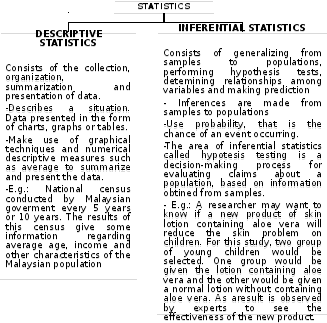# Chapter 3: intoduction to statistics the Statistical Process

 SHF1124 CHAPTER 3: INTODUCTION TO STATISTICS The Statistical ProcessPlan the Investigation: What? How? Who? Where? Collect the Sample Collect 3.1 Introduction Statistics: A field of study which implies collecting, presenting, analyzing and interpreting data as a basis for explanation, description and comparison. used to analyze the results of surveys and as a tool in scientific research to make decisions based on controlled experiments. Also useful for operations, research, quality control, estimation and prediction. Population: a collection, or set of individuals or objects or events whose properties are to be analyzed. Sample: a group of subjects selected from the population. Sample is a subset of a population. Data: consist a set of recorded observations or values. Any quantity that can have a number of values is variable. Variables whose values are determined by chance are called random variables. Data set: a collection of data values. Each value in the data set is called a data value or a datum. Variable: a characteristics or attribute that can assume different values. A statistical exercise normally consists of 4 stages: Collection of data by counting or measuring. Ordering and presentation of the data in a convenient form. Analysis of the collected data. Interpretation of the results and conclusions formulated.Download 341.52 Kb.Share with your friends: